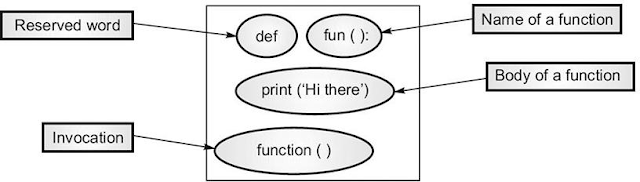## Posts

Showing posts with the label function

### Swap Two Strings in Python Perfect Way Using FunctionYou can swap Two Strings  in Python without the need to define the third variable. You can achieve this with function. Here's an example for your practice. Python ideas How to Swap Two Strings You can use multiple arguments in the function. Here, I've given 'a' and 'b' as arguments for the swap function. When you use the swap function, you will get swapped result. def swap(a, b):  return b,a  Use the above function to swap Strings. i = "Hello world" j = "This is ApplyBigAnalytics"  (i, j) = swap(i, j)  print(i)  print(j)  Example to Swap Two Numbers. i = 1  j = 2  (i, j) = swap(i,j)  print(i)   print(j) The Result is: This is ApplyBigAnalytics  Hello world  2  1 Keep Reading The Real Purpose of Underscore in Python

### Python How to Create FunctionIn Python, you can define and call the function. Once done, you can call it later from the other program. I have shared the structure of it. And, I have added the three rules of it; useful for interviews. Structure of Function Structure of Function Rules to Create a Function A function definition is executable. The body will have the code to perform the task you wish. You can assign default parameter values. 1. Key Elements. You will find here three key elements of the Python function. Here you can read the parameter vs argument differences . Name of a function. It can be any valid identifier. It should be meaningful, and it should convey the work it will do. Parameter. These should be separated with command and should be in the parentheses following the name of the function. The parameters are input to function. It can have any parameters. Body of the function. The function body can contain code that implements the task to be performed by it. 2. Sample Function. Python Example. def